# The DISTANCE Procedure

### WEIGHT Statement

WGTWEIGHT variable ;

The WEIGHT statement specifies a numeric variable in the input data set with values that are used to weight each observation. This weight variable is used for standardizing variables rather than computing the distances. Only one variable can be specified.

The WEIGHT variable values can be nonintegers. An observation is used in the analysis only if the value of the WEIGHT variable is greater than zero. The WEIGHT variable applies to variables that are standardized by the following options: STD=MEAN, STD=SUM, STD=EUCLEN, STD=USTD, STD=STD, STD=AGK, or STD=L.

PROC DISTANCE uses the value of the WEIGHT variable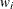to compute the sample mean, uncorrected sample variances, and sample variances as follows: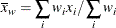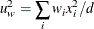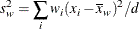is the weight value of the ith observation,is the value of the ith observation, and d is the divisor controlled by the VARDEF= option (see the VARDEF= option in the PROC DISTANCE statement for details).

PROC DISTANCE uses the value of the WEIGHT variable to calculate the following statistics for standardization:

MEAN

the weighted mean,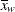SUM

the weighted sum,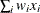USTD

the weighted uncorrected standard deviation,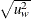STD

the weighted standard deviation,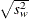EUCLEN

the weighted Euclidean length, computed as the square root of the weighted uncorrected sum of squares: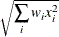AGK

the AGK estimate. This estimate is documented further in the ACECLUS procedure as the METHOD=COUNT option. See the discussion of the WEIGHT statement in Chapter 24: The ACECLUS Procedure, for information about how the WEIGHT variable is applied to the AGK estimate.

L

the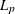estimate. This estimate is documented further in the FASTCLUS procedure as the LEAST= option. See the discussion of the WEIGHT statement in Chapter 38: The FASTCLUS Procedure, for information about how the WEIGHT variable is used to compute weighted cluster means. Note that the number of clusters is always 1.